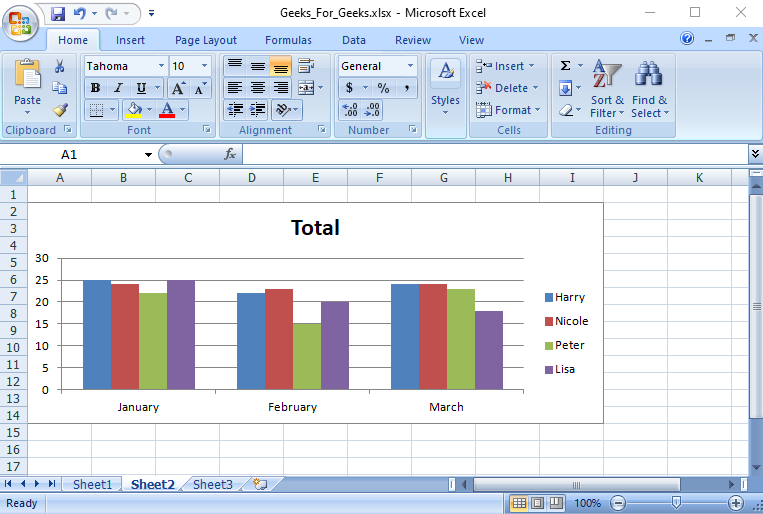GeeksforGeeks App
Open AppBrowser
Continue

# How to Create Pivot Chart from Pivot Table in Excel using Java?

A Pivot Chart is used to analyze data of a table with very little effort (and no formulas) and it gives you the big picture of your raw data. It allows you to analyze data using various types of graphs and layouts. It is considered to be the best chart during a business presentation that involves huge data. To add a pivot chart to an Excel worksheet, you need to use the WorksheetChartsCollection.add method. Before Creating Pivot Chart first needs to go through how to Create Pivot Table in excel using Java. Now let’s discuss steps to create a pivot chart in an Excel file in Java using Free Spire.XLS for Java API.

### Step by Step Implementation

Step 1: Load the Excel file

```Workbook workbook = new Workbook()
String workbookName = "Geeks_For_Geeks.xlsx";

Step 2: Get the first worksheet

`Worksheet sheet = workbook.getWorksheets().get(0);`

Step 3: Get the first pivot table in the worksheet

`IPivotTable pivotTable = sheet.getPivotTables().get(0);`

Step 4: Add a clustered column chart based on the pivot table to the second worksheet

`Chart chart = workbook.getWorksheets().get(1).getCharts().add(ExcelChartType.ColumnClustered, pivotTable);`

Step 5: Set chart position

```chart.setTopRow(2);
chart.setBottomRow(15);```

Step 6: Set chart title

`chart.setChartTitle("Total");`

Step 7: Save the result file

`workbook.saveToFile(workbookName, ExcelVersion.Version2013);`

Let’s write Java Program to create a pivot chart from a pivot table in a spreadsheet.

## Java

 `import` `com.spire.xls.*;``import` `com.spire.xls.core.IPivotTable;`` ` `class` `GFG {``    ``public` `static` `void` `main(String[] args)``    ``{``        ``// Load the Excel file``        ``Workbook workbook = ``new` `Workbook();``        ``String workbookName = ``"Geeks_For_Geeks.xlsx"``;``        ``workbook.loadFromFile(workbookName);`` ` `        ``// Get the first worksheet``        ``Worksheet sheet = workbook.getWorksheets().get(``0``);``       ` `        ``// get the first pivot table in the worksheet``        ``IPivotTable pivotTable``            ``= sheet.getPivotTables().get(``0``);`` ` `        ``// Add a clustered column chart based on the pivot``        ``// table to the second worksheet``        ``Chart chart``            ``= workbook.getWorksheets()``                  ``.get(``1``)``                  ``.getCharts()``                  ``.add(ExcelChartType.ColumnClustered,``                       ``pivotTable);``       ` `        ``// Set chart position``        ``chart.setTopRow(``2``);``        ``chart.setBottomRow(``15``);``       ` `        ``// Set chart title``        ``chart.setChartTitle(``"Total"``);`` ` `        ``// Save the result file``        ``workbook.saveToFile(workbookName,``                            ``ExcelVersion.Version2013);``        ``System.out.println(workbookName``                           ``+ ``" is written successfully"``);``    ``}``}`

Output: On the console window when the program is successfully executed.

`GeeksForGeeks.xlsx is written successfully.`

Output: On the Workbook(excel file)My Personal Notes arrow_drop_up
Related Tutorials